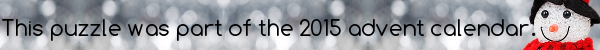mscroggs.co.uk
mscroggs.co.uksubscribe

# Puzzles

## 23 DecemberThis number is a prime number. If you treble it and add 16, the result is also prime. Repeating this will give 11 prime numbers in total (including the number itself).

## 14 DecemberWhat is the only palindromic three digit prime number which is also palindromic when written in binary?

## 3n+1

Let $$S=\{3n+1:n\in\mathbb{N}\}$$ be the set of numbers one more than a multiple of three.
(i) Show that $$S$$ is closed under multiplication.
ie. Show that if $$a,b\in S$$ then $$a\times b\in S$$.
Let $$p\in S$$ be irreducible if $$p\not=1$$ and the only factors of $$p$$ in $$S$$ are $$1$$ and $$p$$. (This is equivalent to the most commonly given definition of prime.)
(ii) Can each number in $$S$$ be uniquely factorised into irreducibles?

## Archive

Show me a random puzzle
▼ show ▼